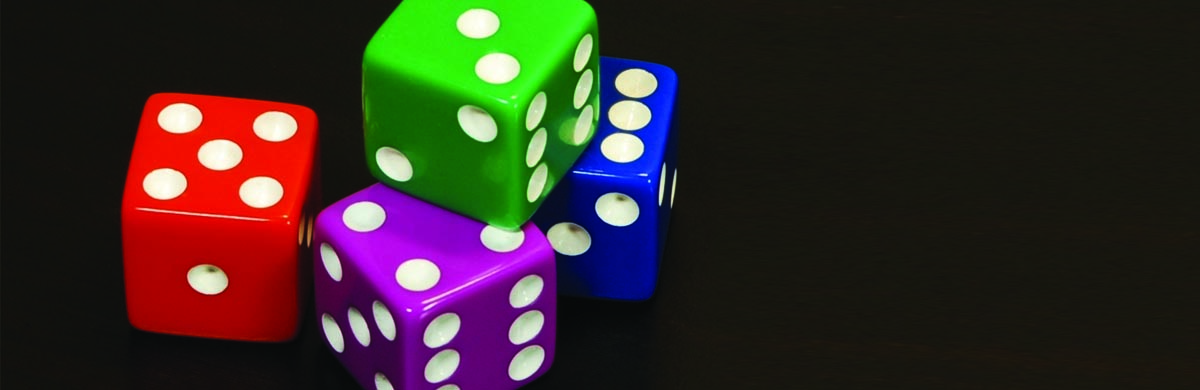# Probability Tip: Three Strategies That Aren’t Used EnoughSome GMAT instructors will say that students often place too much emphasis on studying for Probability and Combinatorics, rather than spending more time focusing on the heavy lifters of Algebra and Arithmetic.

Like it or not, GMAT students get fixated on combinatorics and probability because a) these questions are never, never as straightforward as remembering and plugging into the permutation or combination formula and b) these problems have the illusion of being easy and attainable. But these sticky types of GMAT questions are notorious time wasters, driving test takers deep into a rabbit holes without consideration the right strategies.

In this tip, we take a look at three helpful strategies – inverse probability, “lucky twins,” and guess-timating.

Let’s start with considering approaching the question backwards, or rather, looking at the inverse probability first.

So, what exactly does inverse probability mean? Take this question for example:

Each year, three space shuttles are launched: two in June and one in October. If each shuttle launch is known to occur without a delay in 90% of the cases, and if the current month is January, what is the probability that at least one of the launches in the next 16 months will be delayed?

1. 1/27
2. 3/27
3. 271/1000
4. 729/1000
5. 26/27

This notorious “probability that at least one” question can get even the most diligent students down the road of a messy mathematical mess. What the GMAT would love for you to do is try to figure out every time a delayed launch happens, then divide by the total number of possibilities.

This approach is time-consuming and very prone to error. An easier approach is considering the “inverse probability,” or rather, P(A) = 1 – P(Not A) – the inverse of “at least one” is “none at all,” and “none at all” is easier to understand conceptually and calculate mathematically. Calculate “none at all” and subtract from 1 to get the correct answer of “at least one.”

For this specific question, the answer is found by understanding the chance of having no delays is 90% – so the chance of three consecutive launches without having a delay would be:

90/100 x 90/100 x 90/100 = 729/1000

Smart, time-conscious test takers will fairly quickly realize the answer must be (C) 271/1000 without doing any additional math. Clearly, we want to determine 1 – 729/100, and the units digit in the numerator will most certainly be 1, which would not reduce any further with 1000 as the denominator.

This ties into the second strategy for probability and combinatorics questions – considering “lucky twins,” and guess-timating what an answer might be by understanding what it should be close to based upon available answer choices.

“Lucky twins” are answer choices that add up to 1 in a probability question and can be a consideration if you are running out of time on a complicated problem. If it seems like the situation in the problem happens more often than not, pick the larger of the two lucky twins. If the situation in the problem seems to happen less often, pick the smaller of the two twins, and you might find yourself in lucky with a right answer.

When it comes to guess-timating answer choices, consider doing so on a problem like this one:

20 people at a meeting were born during the month of September, which has 30 days. The probability that at least two of the people in the room share the same birthday is closest to which of the following?

1. 10%
2. 33%
3. 67%
4. 90%
5. 99%

This problem is an impossible nightmare to solve mathematically. Instead, evaluating the answer choices, at the very least every answer choice except for D) and E) should be discounted, giving the test take a 50/50 chance of getting it correct. 20 people and 30 days – when thinking about the inverse probability, the likelihood of not having overlap between birthdays is incredibly small. The correct answer is, however, E).

In summary – try not to be bogged down by complex combinatorics and probability questions. While these do show up less often on the GMAT, overall, evaluate and weigh how the amount of time required to solve one of these problems versus understanding that, with these strategies, you should be able to get to a 50/50 chance quickly, giving yourself more time to solve the questions you do know how to answer.

The above GMAT Tip comes from Veritas Prep. Since its founding in 2002, Veritas Prep has helped more than 100,000 students prepare for the GMAT and offers the most highly rated GMAT Prep course in the industry.